# 利用 Python 爬取了 13966 条运维招聘信息，我得出了哪些结论？JackTian﻿

﻿

﻿

﻿

﻿

﻿

• 热门行业的用人需求 Top10

• 热门城市的岗位数量 Top10

• 岗位的省份分布

• 不同公司规模的用人情况

• 排名前 10 的岗位的平均薪资

• 岗位对学历的要求

• 运维岗位需求的词云图分布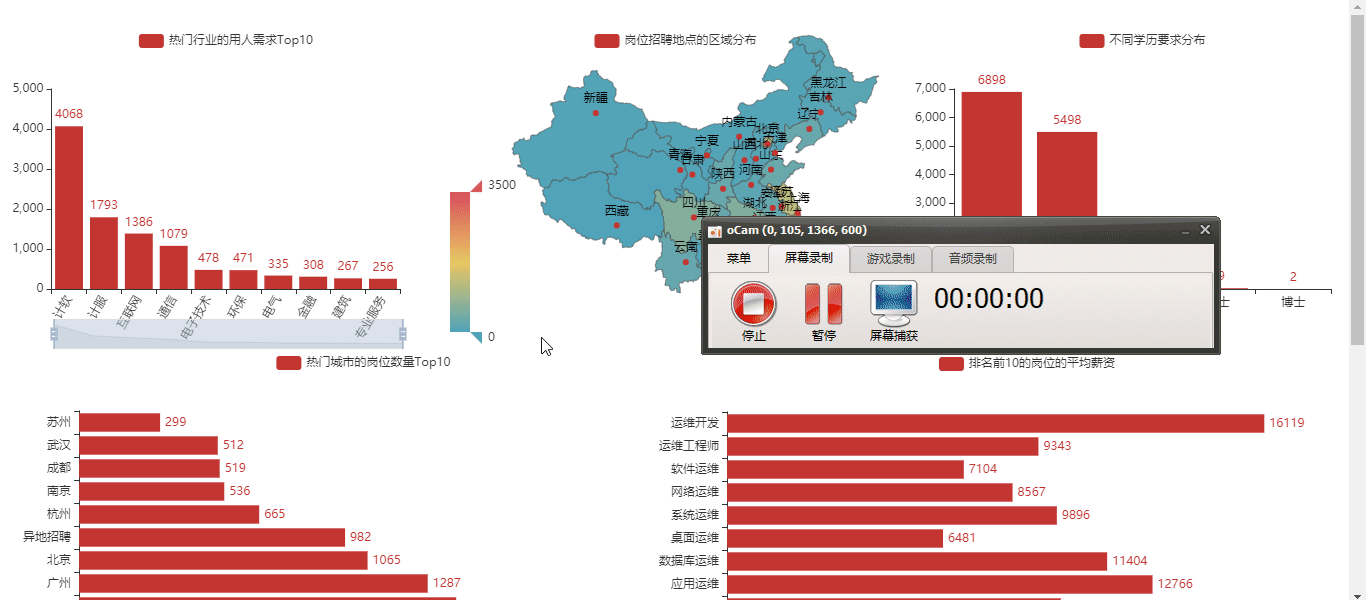﻿

﻿

• 爬虫部分

• 数据清洗

• 数据可视化及分析

#### 1、爬虫部分

﻿

﻿

# 1、岗位名称job_name = dom.xpath('//div[@class="dw_table"]/div[@class="el"]//p/span/a[@target="_blank"]/@title')﻿# 2、公司名称company_name = dom.xpath('//div[@class="dw_table"]/div[@class="el"]/span[@class="t2"]/a[@target="_blank"]/@title')﻿# 3、工作地点address = dom.xpath('//div[@class="dw_table"]/div[@class="el"]/span[@class="t3"]/text()')﻿# 4、工资salary_mid = dom.xpath('//div[@class="dw_table"]/div[@class="el"]/span[@class="t4"]')salary = [i.text for i in salary_mid]﻿# 5、发布日期release_time = dom.xpath('//div[@class="dw_table"]/div[@class="el"]/span[@class="t5"]/text()')﻿# 6、获取二级网址urldeep_url = dom.xpath('//div[@class="dw_table"]/div[@class="el"]//p/span/a[@target="_blank"]/@href')﻿# 7、爬取经验、学历信息，先合在一个字段里面，以后再做数据清洗。命名为random_allrandom_all = dom_test.xpath('//div[@class="tHeader tHjob"]//div[@class="cn"]/p[@class="msg ltype"]/text()')﻿# 8、岗位描述信息job_describe = dom_test.xpath('//div[@class="tBorderTop_box"]//div[@class="bmsg job_msg inbox"]/p/text()')﻿# 9、公司类型company_type = dom_test.xpath('//div[@class="tCompany_sidebar"]//div[@class="com_tag"]/p/@title')﻿# 10、公司规模(人数)company_size = dom_test.xpath('//div[@class="tCompany_sidebar"]//div[@class="com_tag"]/p/@title')﻿# 11、所属行业(公司)industry = dom_test.xpath('//div[@class="tCompany_sidebar"]//div[@class="com_tag"]/p/@title')

#### 2、数据清洗

﻿

1）读取数据

﻿

# 下面使用到的相关库，在这里展示一下import pandas as pdimport numpy as npimport reimport jieba﻿df = pd.read_csv("only_yun_wei.csv",encoding="gbk",header=None)df.head()

2）为数据设置新的行、列索引

# 为数据框指定行索引df.index = range(len(df))# 为数据框指定列索引df.columns = ["岗位名","公司名","工作地点","工资","发布日期","经验与学历","公司类型","公司规模","行业","工作描述"]df.head()

3）去重处理

# 去重之前的记录数print("去重之前的记录数",df.shape)# 记录去重df.drop_duplicates(subset=["公司名","岗位名","工作地点"],inplace=True)# 去重之后的记录数print("去重之后的记录数",df.shape)

4）对岗位名字段的处理

# ① 岗位字段名的探索df["岗位名"].value_counts()df["岗位名"] = df["岗位名"].apply(lambda x:x.lower())﻿# ② 构造想要分析的目标岗位，做一个数据筛选df.shapetarget_job = ['运维','Linux运维','运维开发','devOps','应用运维','系统运维','数据库运维','运维安全','网络运维','桌面运维']index = [df["岗位名"].str.count(i) for i in target_job]index = np.array(index).sum(axis=0) > 0job_info = df[index]job_info.shape﻿job_list = ['linux运维','运维开发','devOps','应用运维','系统运维','数据库运维'            ,'运维安全','网络运维','桌面运维','it运维','软件运维','运维工程师']job_list = np.array(job_list)def rename(x=None,job_list=job_list):    index = [i in x for i in job_list]    if sum(index) > 0:        return job_list[index]    else:        return xjob_info["岗位名"] = job_info["岗位名"].apply(rename)job_info["岗位名"].value_counts()[:10]

5）工资字段的处理

job_info["工资"].str[-1].value_counts()job_info["工资"].str[-3].value_counts()﻿index1 = job_info["工资"].str[-1].isin(["年","月"])index2 = job_info["工资"].str[-3].isin(["万","千"])job_info = job_info[index1 & index2]job_info["工资"].str[-3:].value_counts()﻿def get_money_max_min(x):    try:        if x[-3] == "万":            z = [float(i)*10000 for i in re.findall("[0-9]+\.?[0-9]*",x)]        elif x[-3] == "千":            z = [float(i) * 1000 for i in re.findall("[0-9]+\.?[0-9]*", x)]        if x[-1] == "年":            z = [i/12 for i in z]        return z    except:        return x﻿salary = job_info["工资"].apply(get_money_max_min)﻿job_info["最低工资"] = salary.strjob_info["最高工资"] = salary.strjob_info["工资水平"] = job_info[["最低工资","最高工资"]].mean(axis=1)

6）工作地点字段的处理

address_list = ['北京', '上海', '广州', '深圳', '杭州', '苏州', '长沙',                '武汉', '天津', '成都', '西安', '东莞', '合肥', '佛山',                '宁波', '南京', '重庆', '长春', '郑州', '常州', '福州',                '沈阳', '济南', '宁波', '厦门', '贵州', '珠海', '青岛',                '中山', '大连','昆山',"惠州","哈尔滨","昆明","南昌","无锡"]address_list = np.array(address_list)﻿def rename(x=None,address_list=address_list):    index = [i in x for i in address_list]    if sum(index) > 0:        return address_list[index]    else:        return xjob_info["工作地点"] = job_info["工作地点"].apply(rename)job_info["工作地点"].value_counts()

7）公司类型字段的处理

job_info.loc[job_info["公司类型"].apply(lambda x:len(x)<6),"公司类型"] = np.nanjob_info["公司类型"] = job_info["公司类型"].str[2:-2]job_info["公司类型"].value_counts()

8）行业字段的处理

job_info["行业"] = job_info["行业"].apply(lambda x:re.sub(",","/",x))job_info.loc[job_info["行业"].apply(lambda x:len(x)<6),"行业"] = np.nanjob_info["行业"] = job_info["行业"].str[2:-2].str.split("/").strjob_info["行业"].value_counts()

9）经验与学历字段的处理

job_info["学历"] = job_info["经验与学历"].apply(lambda x:re.findall("本科|大专|应届生|在校生|硕士|博士",x))def func(x):    if len(x) == 0:        return np.nan    elif len(x) == 1 or len(x) == 2:        return x    else:        return xjob_info["学历"] = job_info["学历"].apply(func)job_info["学历"].value_counts()

10）公司规模字段的处理

def func(x):    if x == "['少于50人']":        return "<50"    elif x == "['50-150人']":        return "50-150"    elif x == "['150-500人']":        return '150-500'    elif x == "['500-1000人']":        return '500-1000'    elif x == "['1000-5000人']":        return '1000-5000'    elif x == "['5000-10000人']":        return '5000-10000'    elif x == "['10000人以上']":        return ">10000"    else:        return np.nanjob_info["公司规模"] = job_info["公司规模"].apply(func)

11）将处理好的数据，构造新数据，导出为新的 excel

feature = ["公司名","岗位名","工作地点","工资水平","发布日期","学历","公司类型","公司规模","行业","工作描述"]final_df = job_info[feature]final_df.to_excel(r"可视化.xlsx",encoding="gbk",index=None)

#### 3、数据可视化

﻿

1）可视化大屏效果

﻿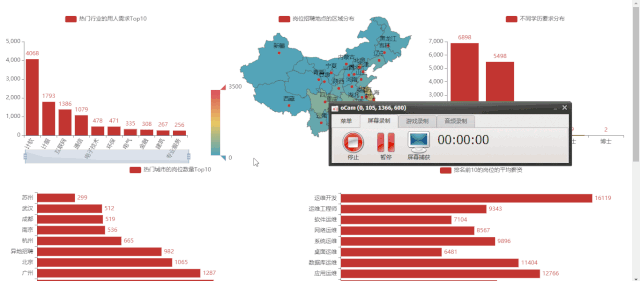2）热门行业的用人需求 Top10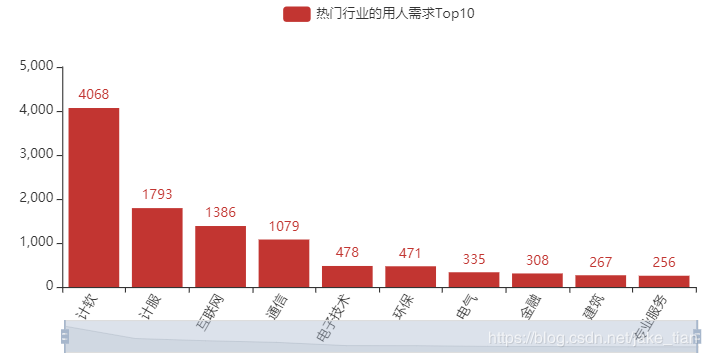3）热门城市的岗位数量 Top10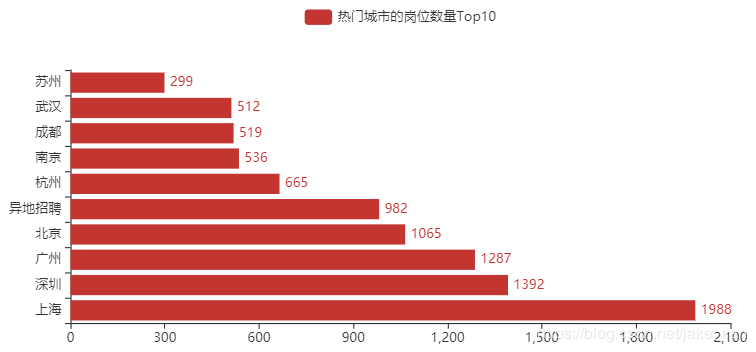4）岗位的省份分布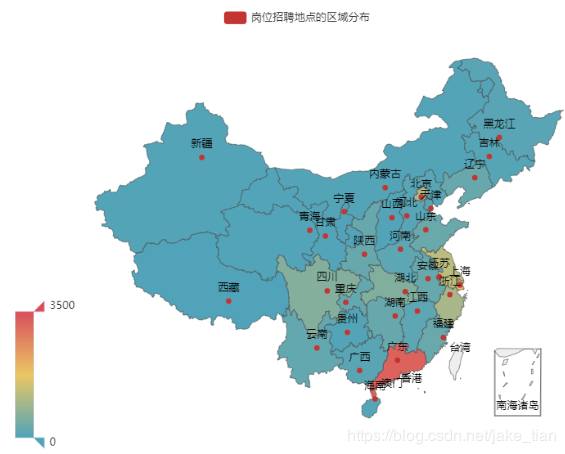5）不同公司规模的用人情况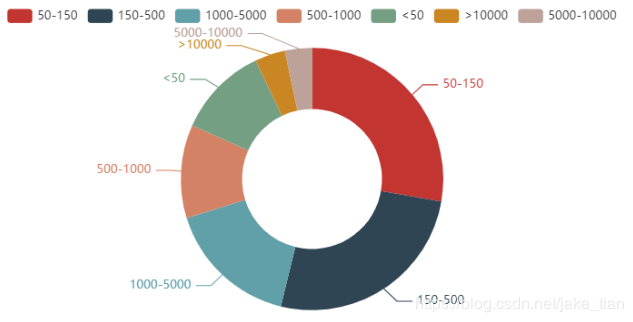6）排名前 10 的岗位的平均薪资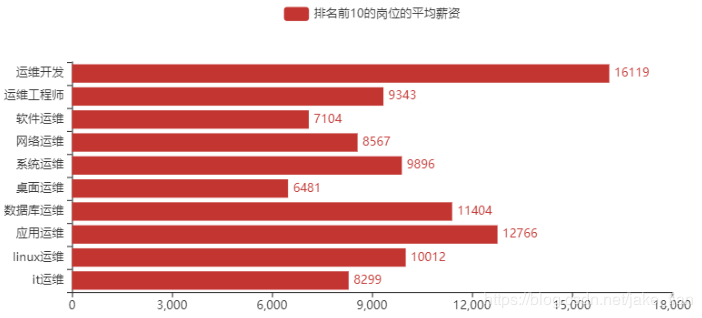﻿

7）运维岗位的学历要求分布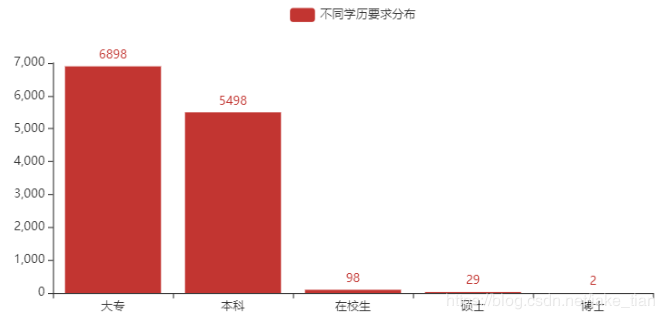8）运维岗位需求的词云图分布#### 总结

﻿

﻿

﻿

﻿

﻿

﻿﻿## 评论